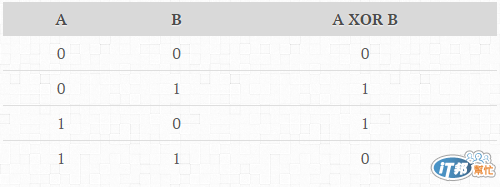#DAY 6
0

## [Day06] 30天挑戰演算法 - 一枝獨秀

[ 1 3 5 7 9 9 7 5 1 ] -> 答案就是 3 (只出現過一次)
[ 1 3 5 7 9 1 7 9 ] -> 不可能會有這種題目，因為題目說了，只有一個數字會是孤單的，其他**保證**都會出現兩次。

XOR 的特色就是 兩兩相同時會抵銷, 舉例來說：
A XOR A XOR B 得到的答案就會是 B
A XOR A XOR B XOR B XOR D XOR C XOR C 得到的答案就是 D
A XOR B XOR A XOR B XOR C 得到的答案就會是 C``````assign array to result
for i in array 1st element to array last element
assign result xor array i into result
return result
``````
• 如果你用 C＃ 可以利用我的 測試程式 來驗證
• 如果你用 Java or Python or C++ 可上 LeetCode Online Judge 驗證
• 還是想不出來嗎？參考我的吧！

Java 先排序，在兩兩相比
public int singleNumber(int[] A) {
Arrays.sort(A);

boolean isFirstHit = true;
int result = -1;
for (int i = 0; i < A.length; i++) {
if (isFirstHit) {
result = A[i];
isFirstHit = false;
} else {
if (result == A[i])
isFirstHit = true;
else
break;
}
}

return result;
}

C# 解答 採用 XOR
public static int SolutionXOR(int[] A)
{
int result = A;
for (int i = 1; i < A.Length; i++)
{
result = result ^ A[i];
}
return result;
}

Python 解答 採用 XOR
def singleNumber(self, A):
if len(A) == 1:
return A

result = A
for i in range(1, len(A), 1):
result = result ^ A[i]
return result

### 3 則留言

0

0